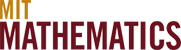# 18.6x

## Probability and Statistics

Many subjects at MIT require acquaintance with probability or statistics, and there are several options for gaining this expertise. The principal ones are 18.05, 6.041, and 18.600. All have 18.02 as a prerequisite. Here is a brief comparison of these three subjects.

• 18.05 (Introduction to Probability and Statistics) is an elementary introduction to both probability and statistics with applications.
• 6.041 (Introduction to Probability) is an introduction to probability theory, and modeling and analysis of probabilistic systems, which also includes a treatment of the elements of statistical inference.
• 18.600 (Probability and Random Variables) covers a broader range of topics in probability, at greater depth than either 18.05 or 6.041, and it is the probability subject of choice for most Mathematics majors. 18.650 is a companion subject on statistics, accepting either 18.600 or 6.041 as prerequisite.